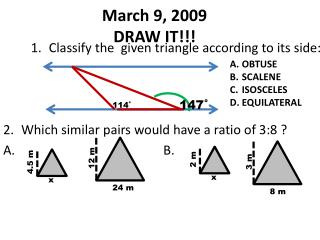DownloadDownload PresentationMarch 9, 2009 DRAW IT!!!

# March 9, 2009 DRAW IT!!!

Télécharger la présentation## March 9, 2009 DRAW IT!!!

- - - - - - - - - - - - - - - - - - - - - - - - - - - E N D - - - - - - - - - - - - - - - - - - - - - - - - - - -
##### Presentation Transcript

1. OBTUSE SCALENE ISOSCELES EQUILATERAL March 9, 2009DRAW IT!!! 147˚ 114˚ Classify the given triangle according to its side: Which similar pairs would have a ratio of 3:8 ? A. B. 4.5 m 2 m 12 m 3 m x x 24 m 8 m

2. 3. Which equation could be used to determine the side length “s” of an isosceles triangle with a perimeter of 50 feet? 17 ft A. B. C. Name the given shape: Isosceles trapezoids have : One pair parallel bases One pair congruent acute angles One pair congruent obtuse angles base base

3. 5. Name the shape: base Right Trapezoids have: One pair parallel bases Two right angles base

4. Where is the base and the height of the figure?

5. NOTES: • PERIMETER OF ANY SHAPE: THE DISTANCE AROUND. ADD ALL SIDES. • CIRCUMFERENCE, REVOLUTION ,ROTATION (FOR CIRCLES USE FORMULA πxd) • RIM • BORDER • EDGE • FRINGE • OUTLINE • FENCE • CAULK • TRIM • TRACE • FRAME • AROUND

6. NOTES: • AREA OF ANY SHAPE: • THE TOTAL INSIDE FLAT SQUARES. • USES base, height,multiplication and sometimes division. • Different formulas for different shapes. • THE HEIGHT MUST FORM A 90° ANGLE! • Mow • Paint • Cover • Carpet • Vacuum • Clean a window • Color • Shade • Floor • Spread • Tile • Squared • Overlay

7. Area = 13² perimeter=13(4) or 52 s = 13 Or 169

8. base = _______ height = ________ Length = ________ Width = _________ Area = __________ perimeter = __________

9. POLYGON QUADRILATERAL PARALLELOGRAMS SQUARES RHOMBUS LEANING SQUARE RECTANGLES PARALLELOGRAMS LEANING RECTANGLE

10. AREA IS THE BASE MULTIPLIED BY THE HEIGHT A = bh